## Finding Edges

This article is part of a series introducing developers to Computer Vision. Check out other articles in this series.

# Finding Edges with the Sobel Operator

In human vision, one of the first steps in comprehending what we see is to learn and understand the outline of things we see.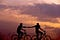We can tell a lot about what is happening in an image by focusing on the edges. (Image Source: Everton Vila)

Edge Detection is an important concept in computer vision where we attempt to extract the outline of objects. We can extract edges from an image when working with kernels and small chunks of pixels. In computer vision, Edges are defined as a region with high-contrast between adjacent pixels — a lighter pixel next to a darker pixel. The larger the difference between adjacent pixels, the higher the contrast and the more defined the edge.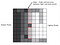Edges are identified by the difference of intensity of neighboring pixels

In the following examples, we are going to be using the grayscale version of following image to explore edges.

Since edges are defined as a change in contrast between adjacent pixels, there’s an inherent directionality when it comes to edges. More simply, are we looking at the difference between adjacent pixels that are on top of each other or side-by-side. Due to this directionality, we construct our Kernels to match edges of a specific orientation. First, we’re going to be looking for changes in contrast between pixels on top of each other — this will result in horizontal edges. The kernel we are using is the Sobel Kernel as shown below:

`[ 1,  2,  1][ 0,  0,  0][-1, -2, -1]`

Looking at the kernel above, we can see how it might emphasize edges in the image. The top side of the kernel is positive and the bottom side of the kernel is negative — emphasizing differences between pixels on top of each other.

Sobel works differently than the blurs we discussed earlier. We are not going to divide by the sum of the kernel values. For now, we’ll just keep the result of the sum operation and use that as interim value.

If any of the results from the convolution is equal to zero, then that means that there is no edge information. We are in a “flat” area in the image. Non-zero results indicates that there is an edge and the further from zero in either positive or negative direction corresponds to harder edge. This is a really simple idea and it shows the power of convolutions!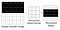When running Sobel through a simplified image, the process is very noticeable!

As you can see in the following image, the horizontal edges have been highlighted. The horizontal edges are more noticeable on the wooden slats that make up the bridge. Notice that information regarding the telephone pole on the left and the wooden siding of the cabin on the right are largely missing. This is because there is very little horizontal change in contrast in these areas.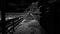The result of the Horizontal Sobel Operator (Normalized) (Original Image Source Simon Migaj)

You probably guessed the Sobel Kernel to extract vertical features from an image is the same as the horizontal kernel, except that it is rotated 90 degrees.

`[-1, 0, 1 ][-2, 0, 2 ][-1, 0, 1 ]`

In the following image we can see that our Sobel Kernel has extracted all of the vertical features in the image. Including the features that were previously absent. And, as we expect, the horizontal features along the bridge is much less noticeable.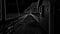The result of the Vertical Sobel Operator (Normalized) (Original Image Source Simon Migaj)

Now, I know what you must be thinking: “What good are two separate images? We want all the edges!” Of course we do. Let’s combine the results of the horizontal and vertical Sobel operations. We have edge information for the horizontal or X direction and we have edge information for the vertical or Y direction, we are able to calculate the final edge information by calculating the hypotenuse (magnitude) of each individual value in the X and Y directions. Once again, we can use the Pythagorean theorem to calculate the magnitude intensity which results in the final pixel value.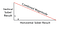We combine the X and Y edges by calculating the magnitude of each pixel value

The result is a single image that highlights edges from all directions.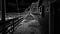The final result of the Sobel Operator (Original Image Source Simon Migaj)

Let’s recap. As with most of the other samples, the first thing we do is convert the image to grayscale. At this point, we run two convolutions through the image: the horizontal Sobel kernel and the vertical Sobel kernel. The result is one set of edge data for the horizontal edges (X edges) and another set of edge data for the vertical edges (Y edges).

Now, we loop through every pixel in the image and for each pixel, we take the corresponding X value from the horizontal edge data and the corresponding Y value from the vertical edge data and we calculate the magnitude.

The magnitude becomes the new pixel value containing both vertical and horizontal edge information.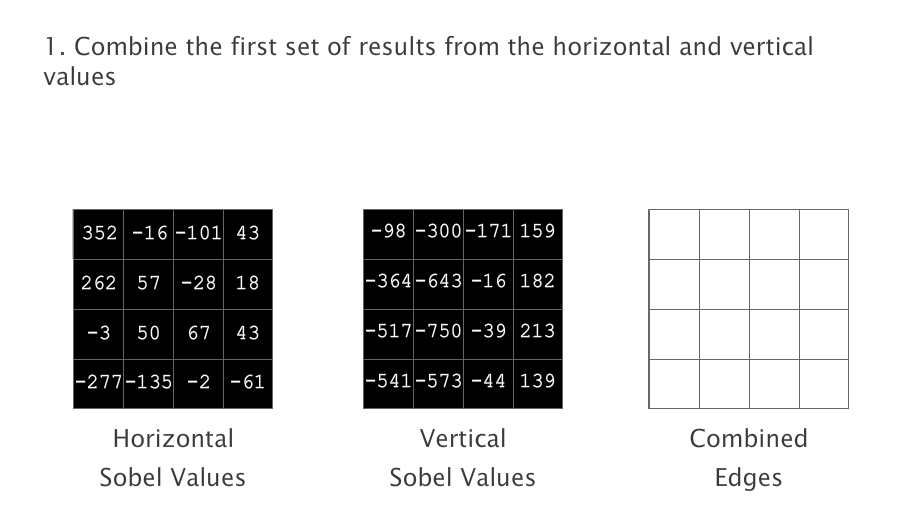Calculate the magnitude of the separate X and Y results to generate the final image with combined edges

As the magnitude is calculated, there is a possibility that the magnitude values may be larger than the capacity of your pixel values. For instance, you can see in the above illustration, we have pixel values greater than our max value of 255. These values can be clamped or they can be scaled/normalized to fit acceptable ranges.

SIDE NOTE: An alternative method of combining the horizontal and vertical Sobel values is to add the absolute values from each. This is a much quicker operation compared to the square root, but is an estimation and may not work well for all use-cases.

The general idea with any edge detection algorithm, is to extract some of the most interesting information from the image. Even after we took away a lot of other information from the above image, most people can still tell what is going on in the photo. The Sobel operator is particularly useful because the results of sobel is often used in other, more advanced algorithms. Developers looking to learn more about edge detection, should also check out the Canny edge detection algorithm which builds on the Sobel operator.

The Sobel Operator has one last magic trick up its sleeve! Due to its structure, the result data contains X and Y values which can be positive or negative — they have a direction. Therefore, we can determine orientation that a particular edge is facing on both the X and Y directions. With this information, we can turn the X and Y values into degrees. This directional information is referred to as the Image Gradient and can be used in other algorithms or used as a way to visualize which direction the edge is facing.

# Image Noise

In the Thresholds and Templates article, we discussed that many computer vision algorithms use grayscale to improve performance and/or reduce some variability. Blurring images is another process which can improve the results of computer vision algorithms. Blurring images can help because it reduces image noise. Noise is a random variation of brightness or color in images. Noise is similar to “grain” in analog cameras. Noise is often visible in dark images where camera sensor attempts to compensate for the lack of light in the environment.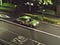An example of a noisy image (Image Source: Ryo Fukasawa)

If we look at the above image carefully, you will notice that the image appears grainy — this grainy look that makes the image appear dirty is Noise.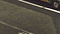Image noise is more noticeable when we zoom into the image (Image Source: Ryo Fukasawa)

Because the Sobel operator looks for changes in contrast between adjacent pixels, the noise will cause our algorithm to report edges where there aren’t any. While Blur kernels are really fun for image processing, they are also very useful in computer vision as they allow us to reduce Noise in images. Using a Box or a Gaussian kernel to blur an image before running the Sobel kernel will reduce the amount of false edges. As we can see in the following images, when we perform a Sobel operator on the original image, our Sobel Kernel picks up the image Noise as edges. Running a simple Box Blur on the image before performing Sobel already cleaned up the image a lot more. Running a blur multiple times can further improve results.

As we can see above, running the Sobel operator on the Noisy Image results in edges being found where there aren’t any — we can see this by the amount of white pixels that show up in the pavement. Running a quick Box Blur filter through the image before running the Sobel Operator makes a huge difference and running the box blur twice greatly improves the quality of edges produced by Sobel.

Running blurs on images is another technique which can help the results of computer vision algorithms.

# TLDR

Understanding edges is very important to human perception and is a huge building block when it comes to Computer Vision. The Sobel Operation is one method of extracting edges from images. The Sobel operation consists of two different kernels. One to find horizontal edges and another to find vertical edges. The results of the two kernels are then combined to exposes all the significant edges in the image. In situations where images are very noisy, it’s a good idea to apply one of the blur filters prior to the Sobel operation to reduce false edges.